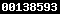### ANALISA GETARAN BEARING BERBASIS VARIASI JARAK PENYANGGA PADA ALAT UJI PUTARAN KRITIS

Abdul Zaki, Budi Hartono, Edi Sutoyo

#### Abstract

This study focused on variation of buffer distance as well as different shaft materials and 3 loading of test specimens. Obtained from the test results on the aluminum shaft at a buffer distance of 60 cm the largest vibration value occurs on the x axis of 17.8 mm/s and the largest deflection occurs at 0.45 m with a weight of 0.135 kg is 0.044 m. Experiment using a buffer distance of 80 cm the largest vibration value occurs on the z axis of 12.6 mm / s and the largest deflection value occurs at 0.6 m with a weight of 0.135 kg is 0.046 m. Experiment using a buffer distance of 100 cm the largest vibration value occurs on the z axis of 14.9 mm/s and the largest deflection occurs at a distance of 0.75 m with a weight of 0.135 kg is 0.088 m. Tests on the stainless steel axle at a buffer distance of 60 cm the largest vibration value occurs on the z axis of 24.1 mm/s and the largest deflection value occurs at a distance of 0.45 m with a weight of 0.135 kg is 0.0037 m. Test uasing a buffer distance of 80 cm the largest vibration value occurs on the x axis of 22.6 mm/s and the largest deflection value occurs at a distance of 0.6 m with a weight of 0.011 kg is 0.046 m. Pada jarak penyangga 100 cm nilai getaran terbesar terjadi pada sumbu z yaitu 14,2 mm/s dan nilai defleksi terbesar terjadi pada jarak 0,75 dengan berat 0,135 yaitu 0,057 m. Testing on the copper shaft at a buffer distance of 60 cm the largest vibration value occurs on the x axis of 20 mm/s and the largest deflection value occurs at a distance of 0.45 m with a weight of 0.135 kg is 0.0032 m. Experiment using a buffer distance of 80 cm the largest vibration value occurs on the x axis of 15 mm/s and the largest deflection value occurs at a distance of 0.6 m with a weight of 0.135 kg is 0.017 m. Test using a buffer distance of 100 cm the largest vibration value occurs on the x axis of 12 mm/s and the largest deflection value occurs at a distance of 0.75 m with a weight of 0.135 kg is 0.119 m.

#### Full Text:

PDF

DOI: http://dx.doi.org/10.32832/ame.v4i1.986

#### Article Metrics

Abstract view : 356 times
PDF - 242 times

### Refbacks

• There are currently no refbacks.

Copyright (c) 2018 Abdul Zaki, Budi Hartono, Edi SutoyoView MyStat